Courses

# Chapter 5 Dynamics Force Analysis And Flywheel - Theory of Machine, Mechanical Engineering Mechanical Engineering Notes | EduRev

## Mechanical Engineering : Chapter 5 Dynamics Force Analysis And Flywheel - Theory of Machine, Mechanical Engineering Mechanical Engineering Notes | EduRev

The document Chapter 5 Dynamics Force Analysis And Flywheel - Theory of Machine, Mechanical Engineering Mechanical Engineering Notes | EduRev is a part of the Mechanical Engineering Course Mechanical Engineering SSC JE (Technical).
All you need of Mechanical Engineering at this link: Mechanical Engineering

Dynamic forces are associated with accelerating masses.

•   D' Alembert's principle states that the inertia forces and couples, and the external forces and torques on a body together give statical equilibrium.
•  Inertia is a properly of matter by virtue of which a body resists any change in velocity.

Inertia force, F1 = – m fg
m = mass of body
fg = acceleration of centre of mass of the body

•   Inertia couple resists any change in the angular velocity  Inertia force, Ci = - Ig. a

Ig = moment of inertia about an axis passing through centre of mass G and perpendicular to plane of rotation of the body
a = angular acceleration of the body.

•  Let F1, F2, F3 etc. = external forces on the body

Tg1, Tg2, Tg3 etc. = external torques on the body about centre of mass G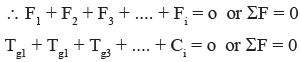Thus a dynamic analysis problem is reduced to one requiring static analysis.

•  The perpendicular displacement h of the force from centre of mass is such that the  torque so produced is equal to the inertia couple acting on the body.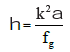where k = radius of gyration
Velocity And Acceleration of Piston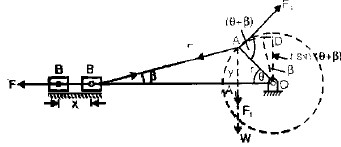If the crank OA rotates in the clockwise direction
l and r = lengths of connecting rod and crank
Let, × = displacement of piston from inner dead centre.
θ = angle turned by crank from inner dead centre.
n = l/r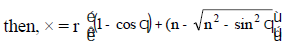then maximum value of sin2q can be unity, and (n>>1), for large connecting rod.
So × = r (1 – cosθ)
This is the expression for a simple harmonic motion. Thus the Piston executes a simple harmonic motion when the connecting rod is large.

•  Velocity of Piston,
v =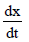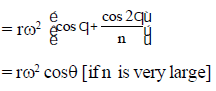•  Angular velocity and Angular Acceleration of connecting rod: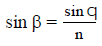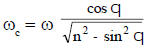•  Angular acceleration of connecting rod: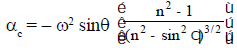The negative sign indicates that the sense of angular acceleration of the rod is such that it tends to reduce the angle b.
Piston Effort (Effective Driving Force)

•   In reciprocating engines, the reciprocating masses accelerate during the first half  of the stroke and the inertia force due to the same tends to resists the motion.  Thus net force on the piston decreased.
•   During the later half of the stroke, the reciprocating masses decelerate and the  inertia force opposes this deceleration or the inertia force acts in the direction of  the applied gas pressure and thus increase the effective force on the piston.
•  In a vertical engine, the weight of the reciprocating masses assists the piston during  the out stroke (down stroke), thus increasing the piston effort by an amount equal  to the weight of the piston.
•  During the in stroke (upstroke) piston effort is decreased by the same amount.

Let, A1 = area of Piston (cover end)
A2 = area of piston end
p1 = pressure on cover end
p2 = pressure on piston end
m1 = mass of reciprocating parts
Force on piston Fp = p1A1 – p2A2
Inertia force; Fb = mf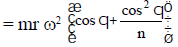Net force on piston F = Fp – Fb

•  Crank Effort: Crank effort is the net effort (force) applied at the crank pin  perpendicular to the crank which gives the required turning moment on the crank  shaft.

Let, Ft = crank effort
Fc = force on connecting rod
F= Fc sin (θ + β)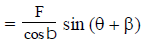•  Turning Moment on Crankshaft

T = Ft × r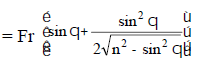•  Inertia of connecting rod: As the motion of connecting rod is not linear.

The mass of connecting rod can be replaced by two point masses at two point, if it is ensured that the two masses together have the same dynamical properties as before.
FLYWHEEL
Function

•  A flywheel used in machines serve as a reservoir, which stores energy during the  period when supply of energy is more than the requirement, and release it during  the period when the requirement of energy is more than the supply. Flywheel does  not maintain a constant speed, it simply reduce fluctuation of speed.

Turning Moment Diagram

•  The turning moment diagram is the graphical representation of the turning moment  or crank-effort. The turning moment is taken as ordinate and crank angle as  abscissa.

Turning Moment diagram for single cylinder four stroke engine

•   In case of a four-stroke internal combustion engine, the diagram repeats itself  after every two revolution instead of one revolution as for a steam engine.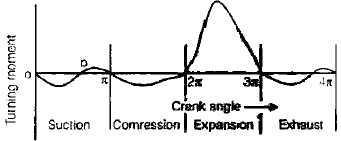•  The pressure inside the engine cylinder is less than the atmoshperic pressure  during the suction stroke, therefore a negative loop is formed.
•  During the compression stroke, the work is done on the gases, therefore a higher negative loop is obtained.
•  During the expansion or working stroke, the fuel burns and the gases expand,  therefore a large positive loop is obtained. In this stroke, the work is done by  the gases.
•  During exhaust stroke, the work is done on the gases, therefore a negative  loop is formed.

T-M diagram for multi-cylinder engine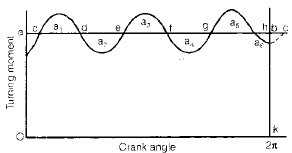•  The turning moment diagram for a single cylinder, engine varies considerably and  a greater variation of the same is observed in case of a four stroke, single cylinder  engine. For engines with more than one cylinder, the total crank shaft torque at  any instant is given by the sum of the torques developed by each cylinder at the  instant.

Fluctuation of Energy

•  The energies of the flywheel corresponding to positions of the crank are as follows: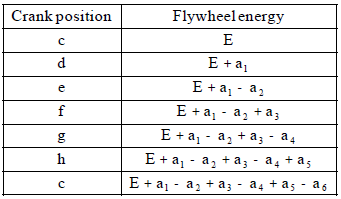From the two values of the energies of the flywheel corresponding to the position c, it is concluded that
a1 – a2 + a3 – a4 + a5 – a6 = 0

•  The greatest of these energies is the maximum kinetic energy of the flywheel and  for the corresponding crank position, the speed is maximum.
•  The least of these energies is the least kinetic energy of the flywheel and for the  corresponding crank position, the speed is minimum.

Fluctuation of Speed

•  The difference between the maximum and minimum speeds during a cycle is called  maximum fluctuation of speed.
•  The ratio of maximum fluctuation of speed to the mean speed is called coefficient of fluctuation of speed (Cs).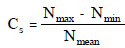•  Coefficient of Steadiness: The reciprocal of the coefficient of fluctuation of  speed is known as coefficient of steadiness and is denoted by m.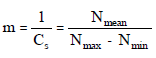Maximum fluctuation of speed
∴ Maximum fluctuation of energy
DE = Maximum energy – Minimum energy
We can also write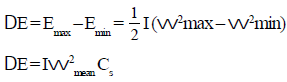Here, I = Mass moment of inertia of the flywheel about its axis of
rotation
wmax = Maximum angular speed during cycle
wmin = Maximum angular speed during cycle
wmean =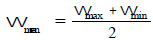during cycle

• Coefficient of Fluctuation of Energy (CE)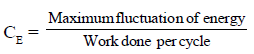Work done per cycle
Work done per cycle = Tmean × q
Work, Tmean is mean torque,
q is angle turned is one cycle
= 2p, in case of two stroke engine
= 4p, in case of 4 stroke engine

Offer running on EduRev: Apply code STAYHOME200 to get INR 200 off on our premium plan EduRev Infinity!

83 docs|53 tests

,

,

,

,

,

,

,

,

,

,

,

,

,

,

,

,

,

,

,

,

,

,

,

,

;